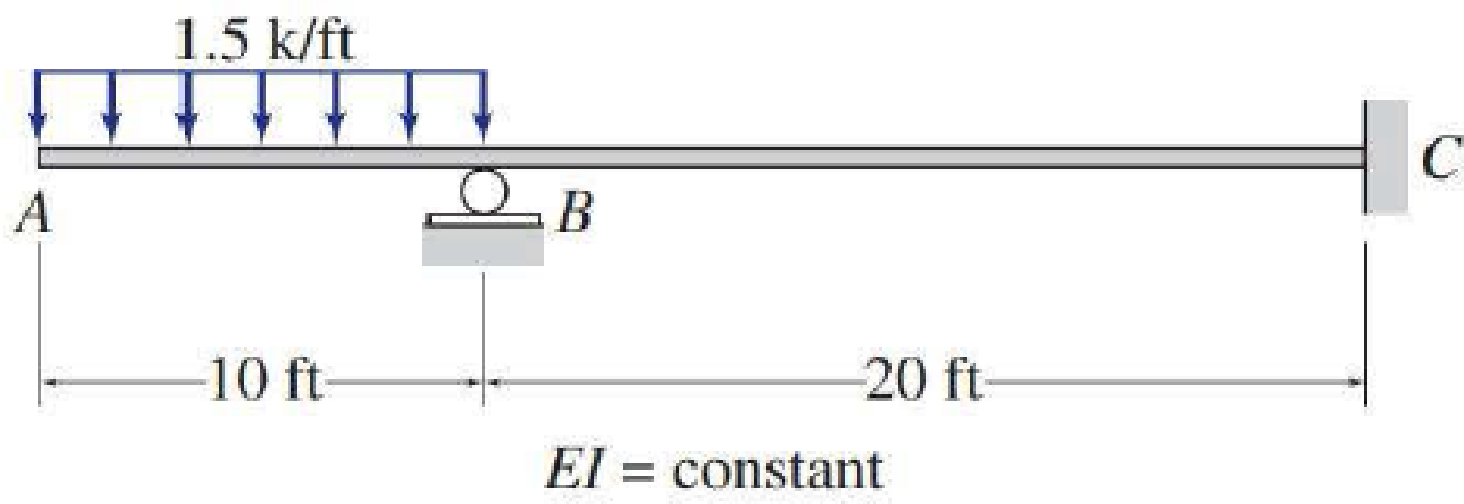# 13.13 through 13.25 Determine the reactions and draw the shear and bending moment diagrams for the structures shown in Figs. P13.13–P13.25 using the method of consistent deformations. FIG. P13.14

#### Solutions

Chapter
Section
Chapter 13, Problem 14P
Textbook Problem
27 views

## 13.13 through 13.25 Determine the reactions and draw the shear and bending moment diagrams for the structures shown in Figs. P13.13–P13.25 using the method of consistent deformations.FIG. P13.14To determine

Find the reactions and sketch the shear and bending moment diagrams for the given beam using method of consistent deformation.

### Explanation of Solution

Given information:

Apply the sign conventions for calculating reactions, forces and moments using the three equations of equilibrium as shown below.

• For summation of forces along x-direction is equal to zero (Fx=0), consider the forces acting towards right side as positive (+) and the forces acting towards left side as negative ().
• For summation of forces along y-direction is equal to zero (Fy=0), consider the upward force as positive (+) and the downward force as negative ().
• For summation of moment about a point is equal to zero (Matapoint=0), consider the clockwise moment as negative and the counter clockwise moment as positive.

Calculation:

Show the free body diagram beam as shown in the Figure 1.

Refer Figure 1.

Consider the horizontal and vertical reaction at C are denoted by Cx and Cy.

Consider the moment at C is denoted by MC.

The reactions acting in the beam is 4.

The number of Equilibrium reaction is 3.

The degree of indeterminacy of the beam is 1.

Take the reaction at B as the redundant.

Modify the Figure 1 as shown in Figure 2.

Refer Figure 2.

The deflection at B due to the external loading is denoted by ΔBO.

The flexibility coefficient representing the deflection at B due to unit value of redundant By is fBB.

Calculate the value of ΔBO as follows:

ΔBO=1EI[0101.5x22](0)dx+1EI[10301.5×10(x5)((x10))]dx=1EI[10301.5×10(x10)(x5)]dx=1EI[103015x2225x+750]dx=(15×x33225×x22+750x)1030

ΔBO=(5x3112.5x2+750x)1030=[(5×303112.5×302+750×30)(5×103112.5×102+750×10)]=55,000EIkft3        (1)

Calculate the value of fBB as follows:

fBB=1EI1030((x10))2dx=1EI1030(x2+10020x)dx=1EI(x33+100</

### Still sussing out bartleby?

Check out a sample textbook solution.

See a sample solution

#### The Solution to Your Study Problems

Bartleby provides explanations to thousands of textbook problems written by our experts, many with advanced degrees!

Get Started

Find more solutions based on key concepts
How do you input elements of a matrix in MATLAB?

Engineering Fundamentals: An Introduction to Engineering (MindTap Course List)

Compute the nominal flexural strength of the composite beam of Problem 9.1-1. Use Fy=50 ksi.

Steel Design (Activate Learning with these NEW titles from Engineering!)

What tool is used to true and dress an aluminum oxide wheel?

Precision Machining Technology (MindTap Course List)

What are the five categories recognized as integral literacy components? (561)

Enhanced Discovering Computers 2017 (Shelly Cashman Series) (MindTap Course List)

Why would a systems analyst have to act as a translator? What groups might be involved?

Systems Analysis and Design (Shelly Cashman Series) (MindTap Course List)

What is a Tier I ERP software vendor?

Fundamentals of Information Systems

Sketch and briefly describe Leavitts diamond.

Principles of Information Systems (MindTap Course List)

What is the difference between law and ethics?

Principles of Information Security (MindTap Course List)

If your motherboard supports ECC DDR3 memory, can you substitute non-ECC DDR3 memory?

A+ Guide to Hardware (Standalone Book) (MindTap Course List)

How far away should highly combustible materials be from any welding or cutting?

Welding: Principles and Applications (MindTap Course List)